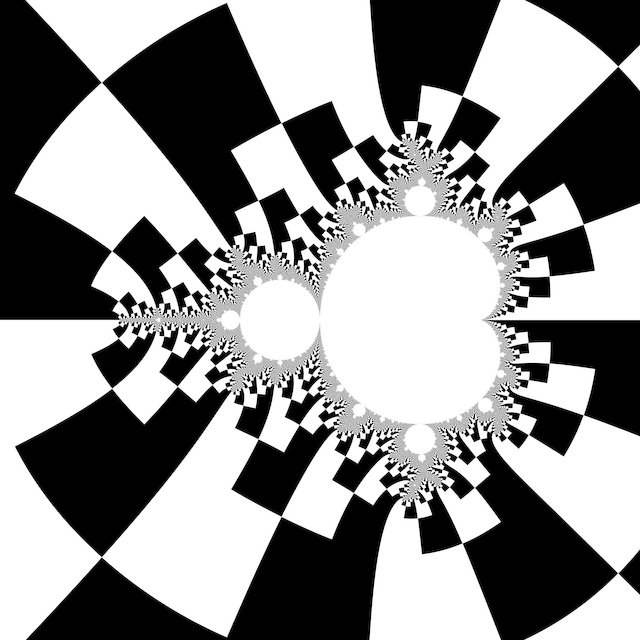# # Binary DecompositionColour according to the sign of $$\Im z$$ (the first escaped iterate). Increasing escape radius is necessary to align edges between layers. Escape radius around $$R = 25$$ makes the decomposed cells roughly square.

## # 1 C99 Code

#include <complex.h>
#include <stdbool.h>

bool m_binary_decomposition(int N, double R, double _Complex c)
{
double _Complex z = 0;
for (int n = 0; n < N; ++n)
{
if (cabs(z) > R)
return cimag(z) > 0;
z = z * z + c;
}
return true;
}